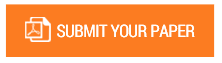The Circle-Line Constant

Authors : Shivam jha

Volume/Issue : Volume 7 - 2022, Issue 5 - May

DOI : https://doi.org/10.5281/zenodo.6547892

Circle-line constant, one of the hidden constant in circle till now. Basically it is the ratio of the radius of a circle to a line whose end points are present in the middle of another line ( the end points of this line are present at the end of a circle’s 1/4th circumference [see fig I]) and middle of the 1/4th circle’s circumference (see fig I). Since, no one has worked on this constant and that’s why this paper will introduce a new constant in mathematics. Material and methods: In this simple mathematical paper work study, a right-angled triangle was drawn. From the middle of the hypotenuse of that triangle to the middle of the circle’s 1/4th circumference, a line was drawn named (k). This distance (k) was directly proportional to the radius (r) of the circle, if we convert 1/4th circumference into a complete circle [see fig II]. Results: Whenever and whatever length of the circle was drawn, its radius to distance (k) ratio was (3.3----). And this result shows us that a constant can be used to remove that proportionality. Conclusion: To remove the proportionality between radius of the circle and distance (k ) , constant , Φ can be used.

Keywords : Circle-line

#### CALL FOR PAPERS

Paper Submission Last Date
30 - September - 2023